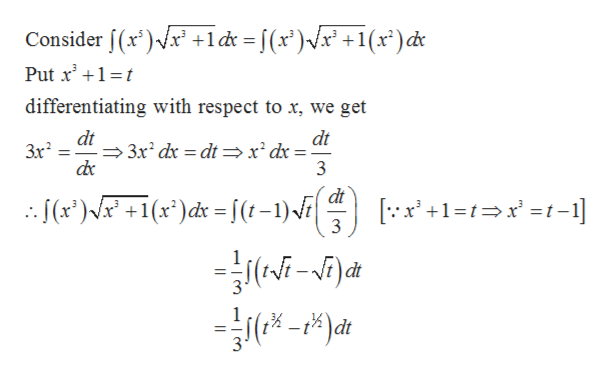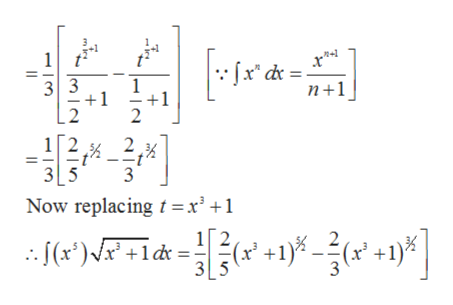Question
35 views

Evaluate the integral.

check_circle

Step 1

Given,

Step 2

Evaluating by substitution:help_outlineImage TranscriptioncloseConsider f(x')/r' +l& = [(x')/x° +1(x*)de Put x' +1=t differentiating with respect to x, we get dt → 3x dx = dt →x² dx = de dt 3x 3 dt : [(x*)/x* +1(x*)dx =[(t-1)\F [:x' +1=t=x' =t-1] 3 ) dt fullscreen
Step 3

Now on integratin...help_outlineImage Transcriptionclose:fx* dx = 3 3 -1 n+1 2 3 5 Now replacing t =x³ +1 : (*)\P +ldt = +1 -? +1%| +1)%. (x³ (r* 3[5 m ini fullscreen

### Want to see the full answer?

See Solution

#### Want to see this answer and more?

Solutions are written by subject experts who are available 24/7. Questions are typically answered within 1 hour.*

See Solution
*Response times may vary by subject and question.
Tagged in
MathCalculus

### Integration